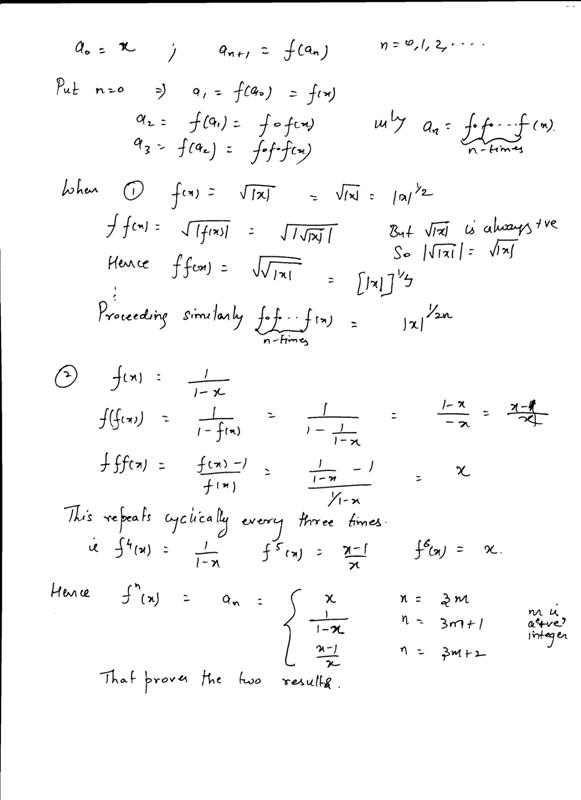# Question:: if a0 = x, an+1 = f(an), n=0,1,2..............               find an when 1) f(x) = square root of mod x 2)    f(x)      =      1/1-x   for convinience answers are 1)mod x1/2n 2)         an    =                   x if n=3m, m is intergers                                      1/1-x if n=3m+1 m is integer                                         x-1/x  if n= 3m+2 mis integer

11 years ago

Hi Shiwani,Hope that helps.

Regards,